# Question 13: How many milliliters of a 0.45 M solution contain 15.0 g of BaCl2? Express...

Question 13:

How many milliliters of a 0.45 M solution contain 15.0 g of BaCl2? Express your answer using two significant figures

Question 14:

A bottle of 12.0 M hydrochloric acid has only 35.4 mL left in it.

A)What will the HCl concentration be if the solution is diluted to 270.0 mL ?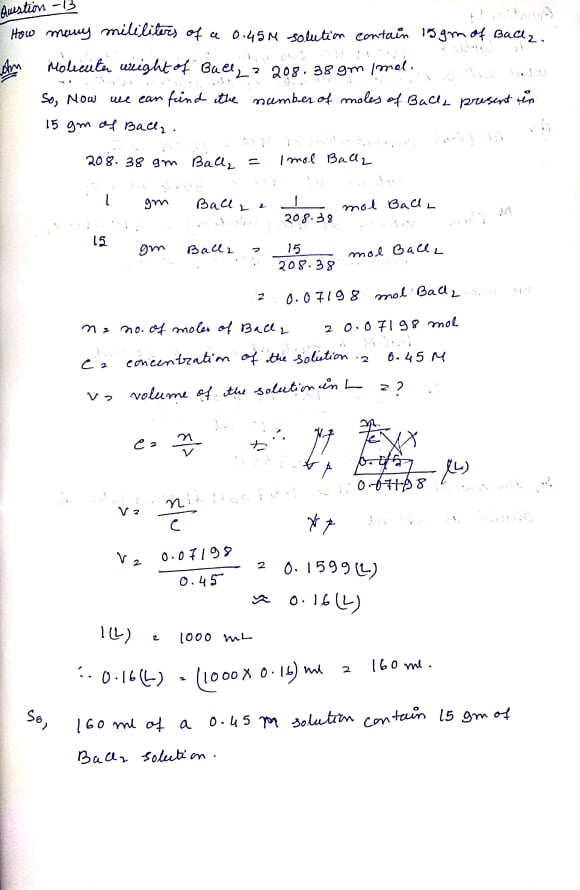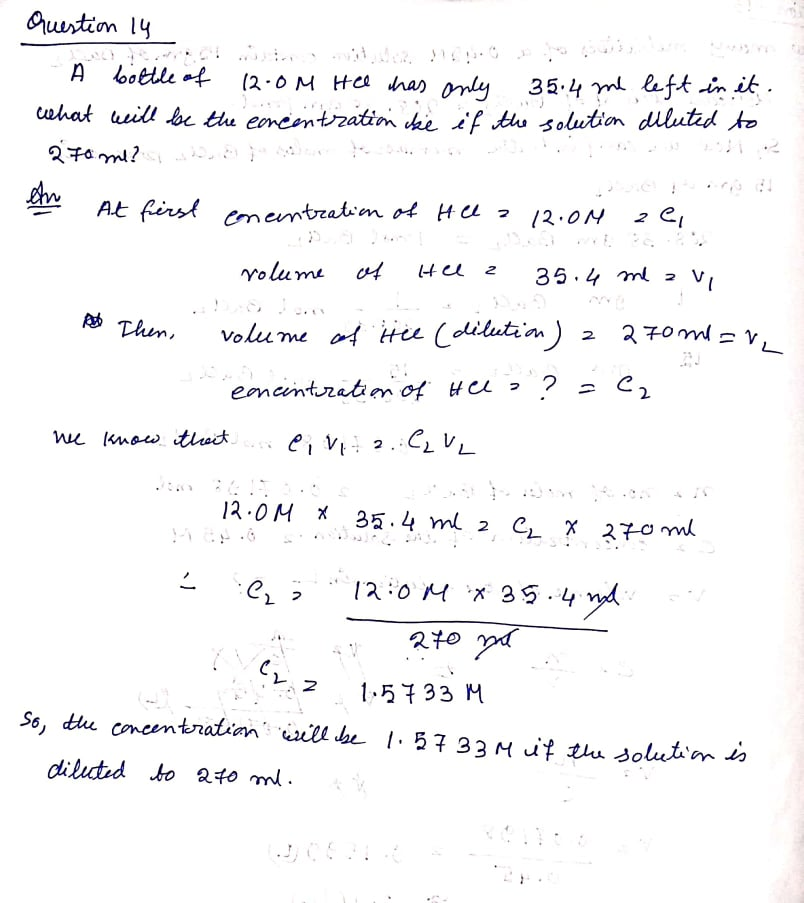#### Earn Coin

Coins can be redeemed for fabulous gifts.

Similar Homework Help Questions
• ### How many milliliters of 0.210 M BaCl2 must be added to 12.4 mL of 0.418 M...

How many milliliters of 0.210 M BaCl2 must be added to 12.4 mL of 0.418 M Na2SO4 to give complete reaction between their solutes? Volume of Ba2+ solution? One of the solids present in photographic film is silver bromide, AgBr. Suppose we want to prepare AgBr by the following precipitation reaction. 2AgNO3(aq) + CaBr2(aq) → 2AgBr(s) + Ca(NO3)2(aq) How many milliliters of 0.123 M CaBr2 solution must be used to react with the solute in 51.1 mL of 0.160 M...

• ### 1. What is the final volume in milliliters when 0.653 L of a 45.4 % (m/v)...

1. What is the final volume in milliliters when 0.653 L of a 45.4 % (m/v) solution is diluted to 24.0 % (m/v)? Express your answer with the appropriate units. 2. A 751 mL NaCl solution is diluted to a volume of 1.06 L and a concentration of 8.00 M . What was the initial concentration? Express your answer with the appropriate units. 3. What volume of 1.00 M HCl in liters is needed to react completely (with nothing left...

• ### (a) How many milliliters of a stock solution of 11.0 M HNO, would you have to...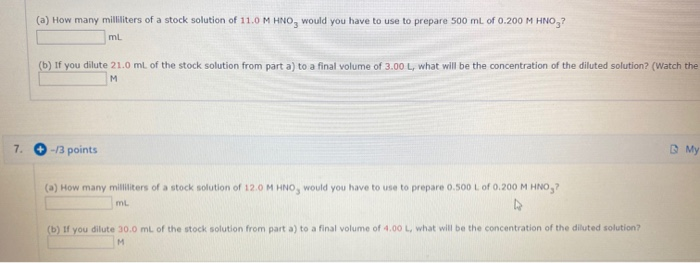(a) How many milliliters of a stock solution of 11.0 M HNO, would you have to use to prepare 500 mL of 0.200 M HNO,? ml (b) If you dilute 21.0 mL of the stock solution from part a) to a final volume of 3.00 L, what will be the concentration of the diluted solution? (Watch the 7. -13 points My (a) How many milliliters of a stock solution of 12.0 M HNO, would you have to use to prepare...

• ### How many milliliters of a 0.390 MKOH solution contain 1.67x10-2 mol of KOH? Express your answer...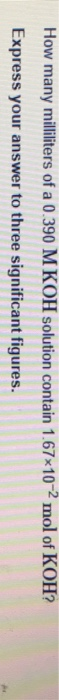How many milliliters of a 0.390 MKOH solution contain 1.67x10-2 mol of KOH? Express your answer to three significant figures.

• ### 28) How many milliliters of a 9.0 M H2504 solution are needed to make 0.45 Lof...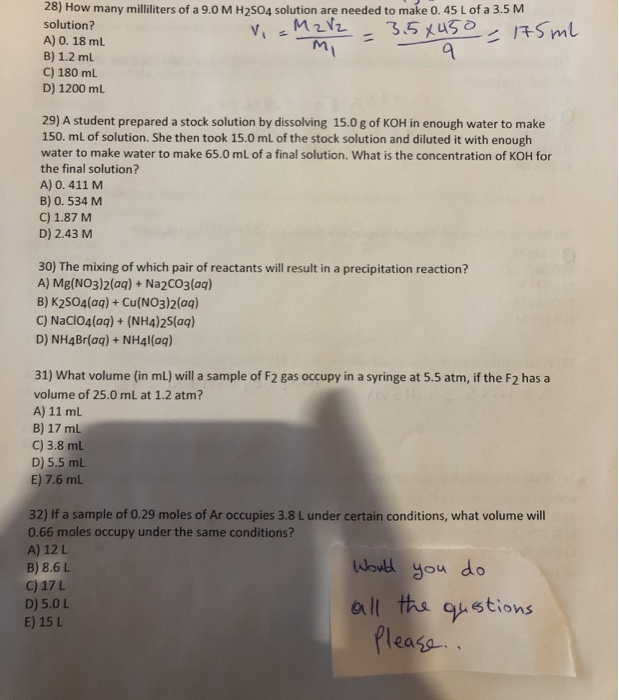28) How many milliliters of a 9.0 M H2504 solution are needed to make 0.45 Lof a 3.5 M solution? Vi=MzV2 - 3.5x450 175ml A) O. 18 ml B) 1.2 ml C) 180 ml D) 1200 ml 29) A student prepared a stock solution by dissolving 15.0 g of KOH in enough water to make 150. mL of solution. She then took 15.0 mL of the stock solution and diluted it with enough water to make water to make 65.0...

• ### Question 13 (2 points) How many milliliters of 1.50 M KOH solution are needed to supply...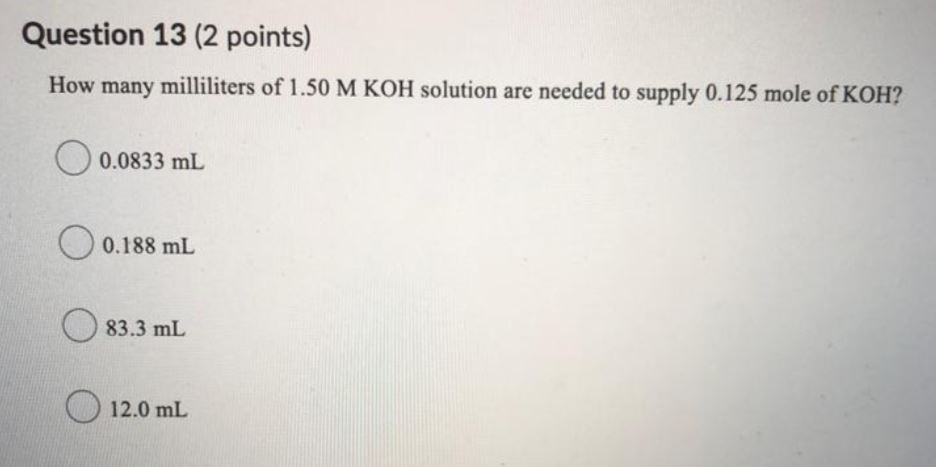Question 13 (2 points) How many milliliters of 1.50 M KOH solution are needed to supply 0.125 mole of KOH? 0.0833 mL 0.188 mL 83.3 mL 12.0 mL

• ### How many milliliters of 10.5 M HCl(aq) are needed to prepare 740.0 mL of 1.00 M...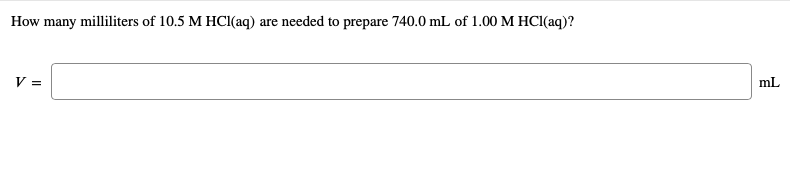How many milliliters of 10.5 M HCl(aq) are needed to prepare 740.0 mL of 1.00 M HCl(aq)? A 61.0 mL aliquot of a 1.20 M solution is diluted to a total volume of 238 mL. A 119 mL portion of that solution is diluted by adding 113 mL of water. What is the final concentration? Assume the volumes are additive. concentration:

• ### How many moles of KMnO4 are present in 125 mL of a 0.0850 M solution? 0.011...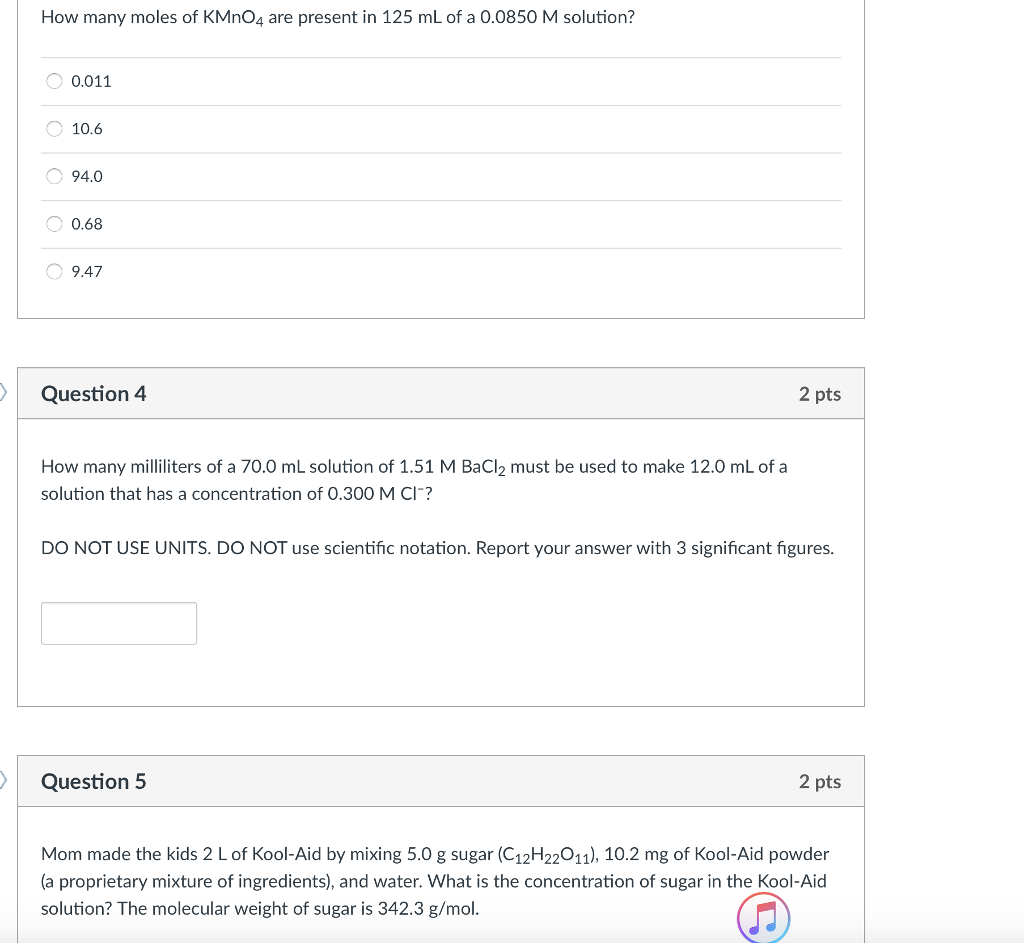How many moles of KMnO4 are present in 125 mL of a 0.0850 M solution? 0.011 10.6 94.0 0.68 9.47 Question 4 2 pts How many milliliters of a 70.0 mL solution of 1.51 M BaCl2 must be used to make 12.0 mL of a solution that has a concentration of 0.300 M CI? DO NOT USE UNITS. DO NOT use scientific notation. Report your answer with 3 significant figures. Question 5 2 pts Mom made the kids 2 L...

• ### 50.0 mL of a stock solution of hydrochloric acid, HCl (aq), at 12.0 M is diluted...

50.0 mL of a stock solution of hydrochloric acid, HCl (aq), at 12.0 M is diluted by adding it to 150.0 mL of water, H2O. What is the concentration, in units of molarity, of the final, diluted solution?

• ### If you had only 23 g of KOH remaining in a bottle, how many milliliters of...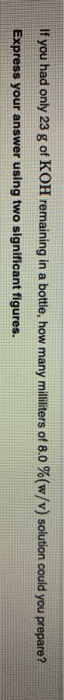If you had only 23 g of KOH remaining in a bottle, how many milliliters of 8.0 %(w/v) solution could you prepare? Express your answer using two significant figures. How many milliliters of 0.27 M solution could you prepare? Express your answer using two significant figures.